Courses

# Chemistry Test 4 - Solution, D And F Block Element, Metallurgy, Alcohol, Phenol And Ether

## 30 Questions MCQ Test JEE Main Mock Test Series 2020 & Previous Year Papers | Chemistry Test 4 - Solution, D And F Block Element, Metallurgy, Alcohol, Phenol And Ether

Description
This mock test of Chemistry Test 4 - Solution, D And F Block Element, Metallurgy, Alcohol, Phenol And Ether for JEE helps you for every JEE entrance exam. This contains 30 Multiple Choice Questions for JEE Chemistry Test 4 - Solution, D And F Block Element, Metallurgy, Alcohol, Phenol And Ether (mcq) to study with solutions a complete question bank. The solved questions answers in this Chemistry Test 4 - Solution, D And F Block Element, Metallurgy, Alcohol, Phenol And Ether quiz give you a good mix of easy questions and tough questions. JEE students definitely take this Chemistry Test 4 - Solution, D And F Block Element, Metallurgy, Alcohol, Phenol And Ether exercise for a better result in the exam. You can find other Chemistry Test 4 - Solution, D And F Block Element, Metallurgy, Alcohol, Phenol And Ether extra questions, long questions & short questions for JEE on EduRev as well by searching above.
QUESTION: 1

Solution:
QUESTION: 2

Solution:
QUESTION: 3

### Among the following, which is least acidic?

Solution:
QUESTION: 4
Which compound is known as oil of winter green ?
Solution:
QUESTION: 5
When phenol is treated with excess bromine water
Solution:
QUESTION: 6
n-Propyl alcohol and iso-propyl alcohol can be chemically distinguished by which reagent
Solution:
QUESTION: 7
In the reaction of phenol with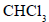and aqueous NaOH at (343 K), the electrophile attacking the ring is
Solution:
QUESTION: 8
Which of the following on oxidation gives a ketone ?
Solution:
QUESTION: 9
Dow’s reaction involves
Solution:
QUESTION: 10
The compound which gives the most stable carbonium ion on dehydration is
Solution:
QUESTION: 11
The compound obtained by the reaction of ethene with diborane followed by hydrolysis with alkaline hydrogen peroxide is
Solution:
QUESTION: 12
When benzenediazonium chloride is treated with water, the compound formed is
Solution:
QUESTION: 13
What amount of bromine will be required to convert 2g of phenol into 2,4, 6-tribromophenol?
Solution:
From the reaction we know that,

Phenol +  3 Br2  --------> 2,4,6-tribromophenol

For 3 Br2 = 3 * (2*80) = 480

Amount of bromine needed  ,

(480*2)/94 = 10.22

((94 is mass of phenol ))

QUESTION: 14

The reaction of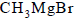with acetone and hydrolysis of the resulting product gives

Solution:
QUESTION: 15
Identify X in the following sequence of reaction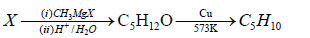Solution:
QUESTION: 16
Which one of the following pairs will not form an ideal solution ?
Solution:
QUESTION: 17
Which of the following pairs of solutions is isotonic ?
Solution:
QUESTION: 18
At 298K, highest osmotic pressure is exhibited by a 0.1M solution of
Solution:
QUESTION: 19
The van’t Hoff factor for a 0.1 M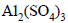solution is 4.20. The degree of dissociation is
Solution:
QUESTION: 20
The molal boiling-point constant for water is 0.513 K/m When 0.1 mole of sugar is dissolved in 200.0 g of water, the solution boils under a pressure of 1.0 atm at
Solution:
QUESTION: 21
When mercuric iodide is added to an aqueous solution of potassium iodide (in excess), a soluble complex,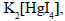is formed. Then,
Solution:
QUESTION: 22

The number of d-electrons in Fe2+ (Z = 26) is not equal to that of

Solution:
QUESTION: 23
Which of the following ions has smallest radii ?
Solution:
QUESTION: 24
The yellow colour of chromates changes to orange on acidification due to formation of
Solution:
QUESTION: 25
The flux used in smelting of copper ores is
Solution: Silica (SiO2) is used in the processes of smelting of copper pyrite (CuFeS2) ore and in conversion of produced copper matte (refined copper sulfide) into a crude metal as a flux (purifying agent).
QUESTION: 26
In the reaction between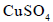and KI, a white precipitate is obtained. The precipitate has the composition
Solution:
QUESTION: 27
An alloy which does not contain copper, is
Solution:
QUESTION: 28
Rust is
Solution:
QUESTION: 29
In the dichromate dianion
Solution:
QUESTION: 30
Arrange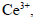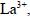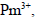and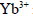in increasing order of their ionic radii
Solution: